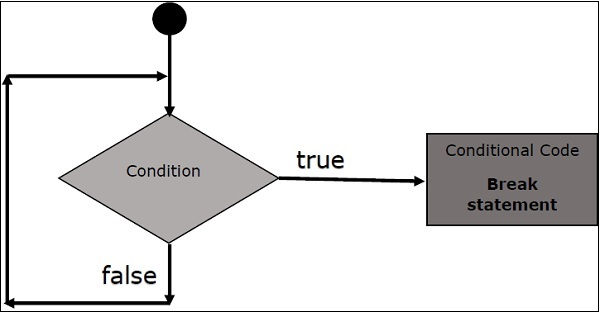# Scala - break Statement

As such there is no built-in break statement available in Scala but if you are running Scala version 2.8, then there is a way to use break statement. When the break statement is encountered inside a loop, the loop is immediately terminated and program control resumes at the next statement following the loop.

## Flow Chart## Syntax

The following is the syntax for break statement.

```// import following package
import scala.util.control._

// create a Breaks object as follows
val loop = new Breaks;

// Keep the loop inside breakable as follows
loop.breakable {
// Loop will go here
for(...){
....

// Break will go here
loop.break;
}
}
```

Try the following example program to understand break statement.

## Example

```import scala.util.control._

object Demo {
def main(args: Array[String]) {
var a = 0;
val numList = List(1,2,3,4,5,6,7,8,9,10);

val loop = new Breaks;

loop.breakable {
for( a <- numList){
println( "Value of a: " + a );

if( a == 4 ){
loop.break;
}
}
}
println( "After the loop" );
}
}
```

Save the above program in Demo.scala. The following commands are used to compile and execute this program.

## Command

```\>scalac Demo.scala
\>scala Demo
```

## Output

```Value of a: 1
Value of a: 2
Value of a: 3
Value of a: 4
After the loop
```

## Breaking Nested Loops

Existing break has an issue while using for nested loops. Just in case to use break for nested loops, follow this method. This is an example program for breaking nested loops.

### Example

```import scala.util.control._

object Demo {
def main(args: Array[String]) {
var a = 0;
var b = 0;
val numList1 = List(1,2,3,4,5);
val numList2 = List(11,12,13);

val outer = new Breaks;
val inner = new Breaks;

outer.breakable {
for( a <- numList1){
println( "Value of a: " + a );

inner.breakable {
for( b <- numList2){
println( "Value of b: " + b );

if( b == 12 ){
inner.break;
}
}
} // inner breakable
}
} // outer breakable.
}
}
```

Save the above program in Demo.scala. The following commands are used to compile and execute this program.

### Command

```\>scalac Demo.scala
\>scala Demo
```

### Output

```Value of a: 1
Value of b: 11
Value of b: 12
Value of a: 2
Value of b: 11
Value of b: 12
Value of a: 3
Value of b: 11
Value of b: 12
Value of a: 4
Value of b: 11
Value of b: 12
Value of a: 5
Value of b: 11
Value of b: 12
```
scala_loop_types.htm